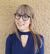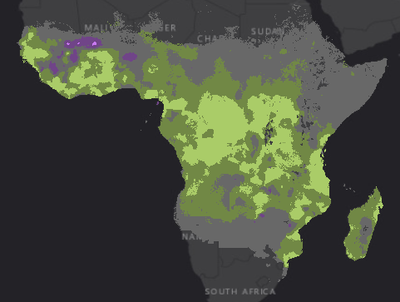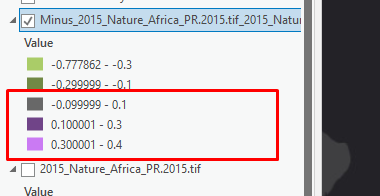# Calculating area using CON (no attribute table)

303
3
02-05-2021 03:33 PM
Labels (3)New Contributor II

Hello there, I'm working on a project where I want to find the area on a map where Malaria rates did not decrease. I can't calculate the area with an attribute table because my map is a continuous dataset.

I'm trying to run CON to find only those areas that meet the criteria vs those that did not, but I don't know how to run the script (I'm a noob on this). I'm attaching two screenshots, basically, I want to calculate the area on my map ONLY where my values are equal and greater than 0.1. I would appreciate your help A LOT1 Solution

Accepted SolutionsbyMVP Frequent Contributor

There's numerous ways to do this, CON will work, but I'd probably just use the reclassify tool (does the same thing really but it's more intuitive for beginners) Reclassify (Spatial Analyst)—ArcGIS Pro | Documentation

first symbolise the data with class breaks of >= 0.1 and <0.1, then drag the layer into that reclassify tool.

Specify >=0.1 as = 1 and <0.1 as NoData, his then produces a new raster of all the raster cells where the value is >=0.1 (with a new value of 1) and applies no data to the rest of the values.

I'd then just create a raster attribute table and count the number of cells, and multiply by the cell size to get the total area.  You could also use raster to polygon and then calculate the area of the polygon (use geodesic area because of the size of your area).

3 RepliesbyMVP Frequent Contributor

There's numerous ways to do this, CON will work, but I'd probably just use the reclassify tool (does the same thing really but it's more intuitive for beginners) Reclassify (Spatial Analyst)—ArcGIS Pro | Documentation

first symbolise the data with class breaks of >= 0.1 and <0.1, then drag the layer into that reclassify tool.

Specify >=0.1 as = 1 and <0.1 as NoData, his then produces a new raster of all the raster cells where the value is >=0.1 (with a new value of 1) and applies no data to the rest of the values.

I'd then just create a raster attribute table and count the number of cells, and multiply by the cell size to get the total area.  You could also use raster to polygon and then calculate the area of the polygon (use geodesic area because of the size of your area).New Contributor II

I can't thank you enough for the help! Perfectly explained, it worked just fine! ♥♥♥byMVP Esteemed Contributor

marked as the Accepted solution

... sort of retired...